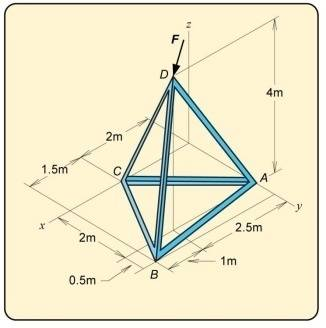# Magnitude of moment about axis (Space Truss)

U1021981

## Homework Statement

Determine the magnitude of the moment of the force F about the axis CA of the tripod
ABCD, if F = {40i -30j -100k}N.

Image attached.

2. The attempt at a solution

Based on a similar question I found in the textbook my method to solve this question:

Find UCA = rCA/rCA = (-2/√8)i + (2/√8)j

Find MCA = UCA.(rDC?? * F)

However, I am unsure if the finding the position vector rDC is correct?

Thanks,

Tim

#### Attachments

•Q6.35.jpg
22.8 KB · Views: 798

Staff Emeritus
Homework Helper
Gold Member

## Homework Statement

Determine the magnitude of the moment of the force F about the axis CA of the tripod
ABCD, if F = {40i -30j -100k}N.

Image attached.

2. The attempt at a solution

Based on a similar question I found in the textbook my method to solve this question:

Find UCA = rCA/rCA = (-2/√8)i + (2/√8)j

Find MCA = UCA.(rDC?? * F)

However, I am unsure if the finding the position vector rDC is correct?